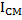# The radius of gyration of a body about an axis at a distance 6 cm from its centre of mass is 10 cm. Then its radius of gyration about a parallel axis through its centre of mass will be in cm is

## Question ID - 52661 :- The radius of gyration of a body about an axis at a distance 6 cm from its centre of mass is 10 cm. Then its radius of gyration about a parallel axis through its centre of mass will be in cm is

3537

From the theorem of parallel axis, the moment of inertiais equal towheremoment of inertia is about centre of mass andthe distance of axis from centre.cm

Next Question :
 The correct  statements among I to II are (I) Valance bond theory cannot explain the color exhibited by transition metal complexes. (II) Valance bond theory can predict quantitatively the magnetic properties of transition metal complexes. (III) Valances bond theory cannot distinguish ligands as weak and strong field ones. (a) (II) and (III) only (b) (I), (II) and (III) (c) (I) and (II) only (d) (I) and (III) only